#### Welcome!

As you explore, keep in mind that every graph in the eBook is the graph of a precise mathematical function!  The graphs do not result from complicated, computer graphics equations or programmed instructions.  Each graph has a clearly defined domain and range, meaning, one number input and one number output.  Even the 3D closed surface graphs have a fourth variable that allows the graph to pass the “vertical line test”!

Imaginary numbers can be defined as running along the depth axis of a 3D coordinate system.

If you’re interested in the rationale for this or just wondering, “Why imaginary numbers”?  Or, “What is an imaginary number?”  See: Imaginary Numbers and Human Experience”.

#### Math eBook

The eBook organizes the various functions with i by the number of dimensions in the function.

#### 3D Functions

A 3D function has three variables.  Each variable is graphed on one of the three space axes.  The functions have one input variable x and two output variables y + iz and are of the form: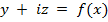The input variable, x, is graphed on the horizontal axis.  The real part of the output variable, y, is graphed on the vertical axis and the imaginary part of the output variable, iz, is graphed on the depth axis.  In this way, the functions produce three dimensional graphs of conics (eBook sec. 2), polynomials (sec. 4), elliptic and hyperelliptic curves (sec. 4.5) and helixes.  Helix functions, in side view, graph dozens of the well-known historical curves (section 3).  A conic 3D function example is below.

#### 4D Functions

A 4D function, of which there are several froms, has four variables. When the 4D function is of the form: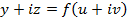the function will graph open surfaces and closed surfaces like the examples in ‘Highlights 3’ and in the gallery images.  These are in eBook sections 12 and 13.

A 4D function of the form: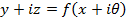combines the rotating natural exponential function with complex natural logarithms. See below the intro to the 6-part blog “Euler’s Formula Upgraded” and eBook section 6.  A variation of these functions generates the “Cardioid Orbits” seen in the slider at the top of this page.  Click on the slider to see the full animation.

Of note, in sections 6 and 11, a 4D function of the form: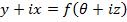generates the rotating imaginary exponential function with complex imaginary logarithms.  The geometric difference between the natural and the imaginary exponential and complex log is here.

Other 4D function forms and their applications: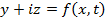algebraic function morphing, sec. 9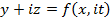helix morphing, sec. 10

#### 5D Functions

5D functions, in eBook sec.14, of the parametric form: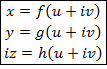will generate circular surfaces like the “triangular torus” and “square torus” seen in the slider at the top of this page.

#### 6D Functions

6D functions, in eBook sec.15, of the parametric form: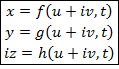will generate closed surface objects in motion like the “Helical Solar System” in the slider at the top of this page.  Click on the slider to see the full animation.  These 6D functions also generate circular surface waves.  One example is in Highlights 3.

#### 3D Function Example

The standard equation of the hyperbola: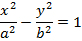with: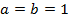And rearranged gives the two functions: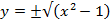with the positive root function in black and the negative root function in red, in three dimensions, this would look like: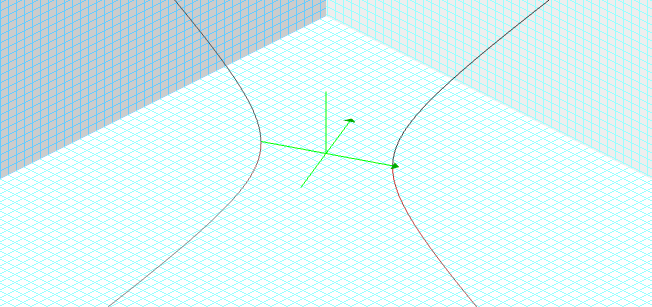Bringing our attention to the area between the vertices, notice that input values: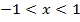will generate imaginary numbers and these are not normally allowed as part of the graph.  When x is in this part of the domain the functions actually generate complex numbers whose real part is zero.   Now that we have a definition for these output imaginary values as being along the depth axis, we can include the domain of x which generates them and graph the two functions as follows.  Positive root in black, negative root in red.

An imaginary circle, in the third dimension, appears between the vertices!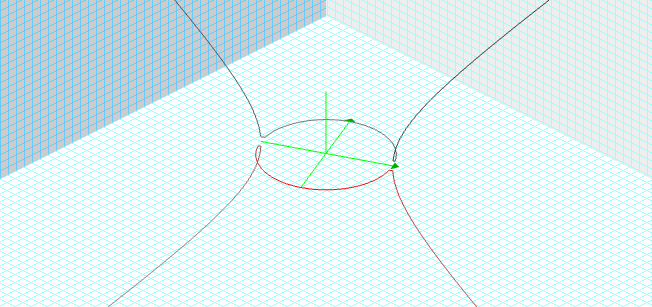(The graphing software has a little trouble at certain vertex points.)

The new coordinate system is called “3Di”, an acronym for Third Dimension Imaginary which makes everything simpler.  The above example and many others are included in the following basic introductory article:

#### A New Geometry of i

part 1:   Elementary Algebraic Functions in 3Di Coordinates

#### Abstract

Imaginary numbers are discussed in relation to human experience and a new coordinate system, “3Di” – an acronym for “Third Dimension Imaginary” is introduced and defined.  A three dimensional function of the form:  [y + iz = f(x)] is then defined.  Polynomials, conics, elliptic curves and hyperelliptic curves are graphed in these coordinates and shown to exist in three dimensions by way of a new and simple geometric definition of an imaginary number.  This definition becomes intuitively obvious and therefore provides a potentially high school level of understanding of complex numbers.  Thus, the new coordinate system itself potentially facilitates a grand enhancement to mathematics education.  The two dimensional functions become three dimensional functions with the notion of a ‘bifurcation point’.  Usually occurring at vertexes, cusps and turning points, a bifurcation point is where multiple branches, both real and imaginary, of the various algebraic functions meet and make various types of turns onto orthogonal planes.

#### Euler’s Formula

Historically and currently Euler’s formula: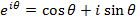enjoys wide agreement as to its algebraic interpretation and meaning.  In this six-part blog we present its many and varied new geometric interpretations and meanings made possible with “3Di” coordinates.

In blog #1 Euler’s formula is used as a rotation of the exponential graph through an imaginary circle.

In blog #2 Euler’s formula is used as an ‘orbit operator’.

In #3 as a helix with both fixed three-dimensional geometry, and in four dimensions with an animation variable.

In #4 we show that Euler’s identity actually maps a point on an ‘exponential/natural log surface’!

In #5 a ‘circular helicoid standing wave’ is shown in which Euler’s imaginary exponential is used no less than five times in the equation: as a surface operator, an orbit operator, a synchronizing rotator, a spin coefficient, and as a reciprocal spin coefficient!

In #6 we present the equation that updates Euler’s formula for all bases: positive, negative, real, imaginary and complex.

“3Di Coordinates” is a completely new concept that makes everything easier to understand. The eBook contains nearly six hundred line and surface graphs illustrating the equations so you can settle in for an enjoyable as well as informative experience.  Thanks!

Sincerely,

greg ehmka

Greg Ehmka’s math e-book demonstrates the real potential of this publishing medium; an active table of contents, an active list of animations, and active cross-referencing make browsing quick and studying delightful. There are also links to external sites that provide instant access to relevant sources. Adding enjoyment and inspiration are over eighty brief videos that animate many of the ground-breaking math equations to colorfully express their resulting objects. This doesn’t begin to speak of the mind-blowing content, which you will just need to see for yourself! Ehmka mingles whimsy with his genius rendering this work both astoundingly brilliant and accessible.

- M.C.W., editor